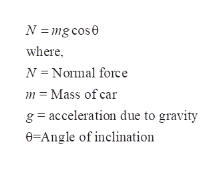# A car is traveling up a hill that is inclined at an angle θ above the horizontal. Determine the ratio of the magnitude of the normal force to the weight of the car when (a)  θ = 16oand (b)  θ = 38o.

Question
652 views

A car is traveling up a hill that is inclined at an angle θ above the horizontal. Determine the ratio of the magnitude of the normal force to the weight of the car when (a)  θ = 16oand (b)  θ = 38o.

check_circle

Step 1

Given:

Step 2

Calculation:

The free body diagram of the car is,

Step 3

The normal force acting on ...help_outlineImage TranscriptioncloseN mg cos where N Nomal force Mass of car m g acceleration due to gravity 6 Angle of inclination fullscreen

### Want to see the full answer?

See Solution

#### Want to see this answer and more?

Solutions are written by subject experts who are available 24/7. Questions are typically answered within 1 hour.*

See Solution
*Response times may vary by subject and question.
Tagged in

### Physics